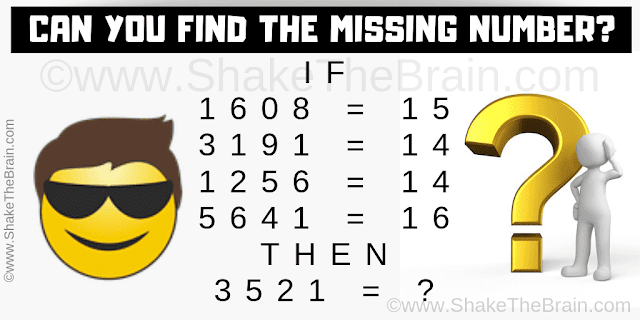## Tuesday, March 3, 2020

Test your Mathematical as well as logical reasoning skills with the Mathematical Logical Brain Teaser. In this Puzzle Picture, there are some logical equations. Can you decode these logical equations and then solve the last equation?Can you solve this mathematical logical brain teaser?

The answer to this "Mathematical Logical Brain Teaser for Teens", can be viewed by clicking on the button. Please do give your best try before looking at the answer.

1.2.3.